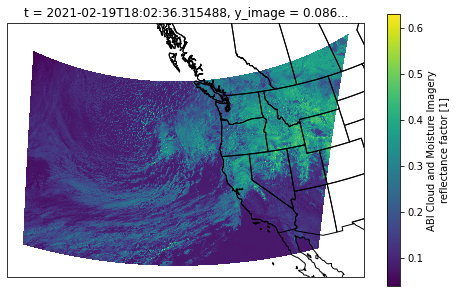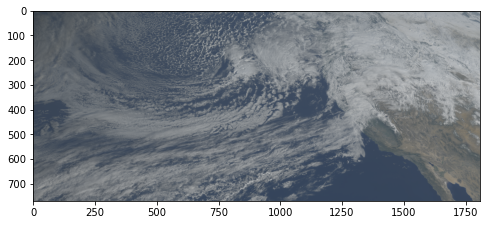# Adding Lat/Lon coordinates to GOES-16/17 L2 Data on Amazon Web Services¶

This tutorial is paired with a medium post that can be found here (and contains more detail on each step).

## Import libraries¶

In :
import xarray as xr
import s3fs
import matplotlib.pyplot as plt
import numpy as np

import cartopy.crs as ccrs
import cartopy.feature as cfeature

In :
import warnings
warnings.filterwarnings('ignore')


## Open file with s3fs and xarray¶

In :
fs = s3fs.S3FileSystem(anon=True)

In :
f = fs.open("s3://noaa-goes17/ABI-L2-MCMIPC/2021/050/18/OR_ABI-L2-MCMIPC-M6_G17_s20210501801176_e20210501803549_c20210501804089.nc")

In :
ds = xr.open_dataset(f)

In :
ds

Out:
<xarray.Dataset>
Dimensions:                                 (band: 1, number_of_image_bounds: 2, number_of_time_bounds: 2, x: 2500, y: 1500)
Coordinates: (12/37)
t                                       datetime64[ns] 2021-02-19T18:02:3...
* y                                       (y) float32 0.1282 ... 0.04427
* x                                       (x) float32 -0.06997 ... 0.06997
y_image                                 float32 0.08624
x_image                                 float32 0.0
band_wavelength_C01                     (band) float32 0.47
...                                      ...
band_id_C11                             (band) int8 11
band_id_C12                             (band) int8 12
band_id_C13                             (band) int8 13
band_id_C14                             (band) int8 14
band_id_C15                             (band) int8 15
band_id_C16                             (band) int8 16
Dimensions without coordinates: band, number_of_image_bounds, number_of_time_bounds
Data variables: (12/124)
CMI_C01                                 (y, x) float32 ...
DQF_C01                                 (y, x) float32 ...
CMI_C02                                 (y, x) float32 ...
DQF_C02                                 (y, x) float32 ...
CMI_C03                                 (y, x) float32 ...
DQF_C03                                 (y, x) float32 ...
...                                      ...
mean_brightness_temperature_C16         float64 260.5
std_dev_brightness_temperature_C16      float64 14.5
percent_uncorrectable_GRB_errors        float64 0.0
percent_uncorrectable_L0_errors         float64 0.0
dynamic_algorithm_input_data_container  int32 -2147483647
algorithm_product_version_container     int32 -2147483647
Attributes: (12/29)
naming_authority:          gov.nesdis.noaa
Conventions:               CF-1.7
standard_name_vocabulary:  CF Standard Name Table (v35, 20 July 2016)
institution:               DOC/NOAA/NESDIS > U.S. Department of Commerce,...
project:                   GOES
...                        ...
date_created:              2021-02-19T18:04:08.9Z
time_coverage_start:       2021-02-19T18:01:17.6Z
time_coverage_end:         2021-02-19T18:03:54.9Z
timeline_id:               ABI Mode 6
production_data_source:    Realtime
id:                        900b3680-135c-454d-a4ac-0940433763d6

## Define function to calculate lat/lon and to dataset as coordinates¶

The math for this function was obtained from https://makersportal.com/blog/2018/11/25/goes-r-satellite-latitude-and-longitude-grid-projection-algorithm

In :
def calc_latlon(ds):
# The math for this function was taken from
# https://makersportal.com/blog/2018/11/25/goes-r-satellite-latitude-and-longitude-grid-projection-algorithm

x = ds.x
y = ds.y
goes_imager_projection = ds.goes_imager_projection

x,y = np.meshgrid(x,y)

r_eq = goes_imager_projection.attrs["semi_major_axis"]
r_pol = goes_imager_projection.attrs["semi_minor_axis"]
l_0 = goes_imager_projection.attrs["longitude_of_projection_origin"] * (np.pi/180)
h_sat = goes_imager_projection.attrs["perspective_point_height"]
H = r_eq + h_sat

a = np.sin(x)**2 + (np.cos(x)**2 * (np.cos(y)**2 + (r_eq**2 / r_pol**2) * np.sin(y)**2))
b = -2 * H * np.cos(x) * np.cos(y)
c = H**2 - r_eq**2

r_s = (-b - np.sqrt(b**2 - 4*a*c))/(2*a)

s_x = r_s * np.cos(x) * np.cos(y)
s_y = -r_s * np.sin(x)
s_z = r_s * np.cos(x) * np.sin(y)

lat = np.arctan((r_eq**2 / r_pol**2) * (s_z / np.sqrt((H-s_x)**2 +s_y**2))) * (180/np.pi)
lon = (l_0 - np.arctan(s_y / (H-s_x))) * (180/np.pi)

ds = ds.assign_coords({
"lat":(["y","x"],lat),
"lon":(["y","x"],lon)
})
ds.lat.attrs["units"] = "degrees_north"
ds.lon.attrs["units"] = "degrees_east"

return ds


## Define function to get x/y bounds given lat/lon bounds¶

In :
def get_xy_from_latlon(ds, lats, lons):
lat1, lat2 = lats
lon1, lon2 = lons

lat = ds.lat.data
lon = ds.lon.data

x = ds.x.data
y = ds.y.data

x,y = np.meshgrid(x,y)

x = x[(lat >= lat1) & (lat <= lat2) & (lon >= lon1) & (lon <= lon2)]
y = y[(lat >= lat1) & (lat <= lat2) & (lon >= lon1) & (lon <= lon2)]

return ((min(x), max(x)), (min(y), max(y)))


In :
ds = calc_latlon(ds)

In :
ds.coords

Out:
Coordinates: (12/39)
t                    datetime64[ns] 2021-02-19T18:02:36.315488
* y                    (y) float32 0.1282 0.1282 0.1281 ... 0.04432 0.04427
* x                    (x) float32 -0.06997 -0.06992 ... 0.06992 0.06997
y_image              float32 0.08624
x_image              float32 0.0
band_wavelength_C01  (band) float32 0.47
...                   ...
band_id_C13          (band) int8 13
band_id_C14          (band) int8 14
band_id_C15          (band) int8 15
band_id_C16          (band) int8 16
lat                  (y, x) float64 53.5 53.49 53.49 ... 14.8 14.8 14.81
lon                  (y, x) float64 -184.4 -184.3 -184.2 ... -112.5 -112.4

## Set lat/lon box and subset the data¶

In :
lats = (30, 55)
lons = (-152, -112)

((x1,x2), (y1, y2)) = get_xy_from_latlon(ds, lats, lons)

In :
subset = ds.sel(x=slice(x1,x2), y=slice(y2, y1))
subset

Out:
<xarray.Dataset>
Dimensions:                                 (band: 1, number_of_image_bounds: 2, number_of_time_bounds: 2, x: 1808, y: 770)
Coordinates: (12/39)
t                                       datetime64[ns] 2021-02-19T18:02:3...
* y                                       (y) float32 0.1282 ... 0.08515
* x                                       (x) float32 -0.03867 ... 0.06252
y_image                                 float32 0.08624
x_image                                 float32 0.0
band_wavelength_C01                     (band) float32 0.47
...                                      ...
band_id_C13                             (band) int8 13
band_id_C14                             (band) int8 14
band_id_C15                             (band) int8 15
band_id_C16                             (band) int8 16
lat                                     (y, x) float64 51.37 51.36 ... 30.01
lon                                     (y, x) float64 -159.1 ... -112.0
Dimensions without coordinates: band, number_of_image_bounds, number_of_time_bounds
Data variables: (12/124)
CMI_C01                                 (y, x) float32 ...
DQF_C01                                 (y, x) float32 ...
CMI_C02                                 (y, x) float32 ...
DQF_C02                                 (y, x) float32 ...
CMI_C03                                 (y, x) float32 ...
DQF_C03                                 (y, x) float32 ...
...                                      ...
mean_brightness_temperature_C16         float64 260.5
std_dev_brightness_temperature_C16      float64 14.5
percent_uncorrectable_GRB_errors        float64 0.0
percent_uncorrectable_L0_errors         float64 0.0
dynamic_algorithm_input_data_container  int32 -2147483647
algorithm_product_version_container     int32 -2147483647
Attributes: (12/29)
naming_authority:          gov.nesdis.noaa
Conventions:               CF-1.7
standard_name_vocabulary:  CF Standard Name Table (v35, 20 July 2016)
institution:               DOC/NOAA/NESDIS > U.S. Department of Commerce,...
project:                   GOES
...                        ...
date_created:              2021-02-19T18:04:08.9Z
time_coverage_start:       2021-02-19T18:01:17.6Z
time_coverage_end:         2021-02-19T18:03:54.9Z
timeline_id:               ABI Mode 6
production_data_source:    Realtime
id:                        900b3680-135c-454d-a4ac-0940433763d6

## Do a quick plot of the blue channel with xarray¶

In :
fig = plt.figure(figsize=(8,5))

p = subset.CMI_C01.plot(
x='lon', y='lat',
subplot_kws={'projection' : ccrs.Orthographic(-130, 40)},
transform=ccrs.PlateCarree()
)


Out:
<cartopy.mpl.feature_artist.FeatureArtist at 0x7f8a507f4f40>## Get RGB data, and calculate "true" green from the "fake" green (veggie) channel¶

In :
r = subset['CMI_C02'].data; r = np.clip(r, 0, 1)
g = subset['CMI_C03'].data; g = np.clip(g, 0, 1)
b = subset['CMI_C01'].data; b = np.clip(b, 0, 1)

In :
gamma = 2.5; r = np.power(r, 1/gamma); g = np.power(g, 1/gamma); b = np.power(b, 1/gamma)
g_true = 0.45 * r + 0.1 * g + 0.45 * b
g_true = np.clip(g_true, 0, 1)
rgb = np.dstack((r, g_true, b))

In :
fig = plt.figure(figsize=(8,5))
plt.imshow(rgb)

Out:
<matplotlib.image.AxesImage at 0x7f8a520b75b0>